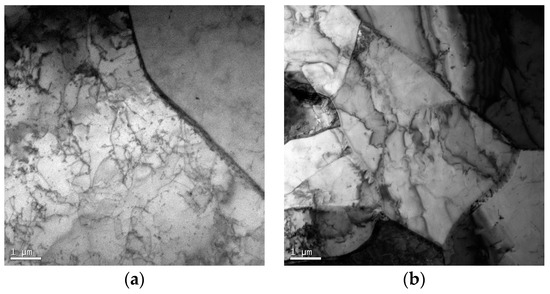Next Article in Journal
Fracture Analysis of MWCNT/Epoxy Nanocomposite Film Deposited on Aluminum Substrate
Previous Article in Journal
Marine Atmospheric Corrosion of Carbon Steel: A Review
Open AccessArticle

# Investigation of a Coupled Arrhenius-Type/Rossard Equation of AH36 Material

byQin Qin 1,*,Ming-Liang Tian 1andPeng Zhang 2
1
School of Mechanical Engineering, University of Science and Technology Beijing, Beijing 100083, China
2
The Tenth System Design Department of the Tenth Research Academy, Guiyang 550009, China
*
Author to whom correspondence should be addressed.
Academic Editors: Richard Thackray and Geminiano Mancusi
Materials 2017, 10(4), 407; https://doi.org/10.3390/ma10040407
Received: 29 December 2016 / Revised: 2 April 2017 / Accepted: 6 April 2017 / Published: 13 April 2017
High-temperature tensile testing of AH36 material in a wide range of temperatures (1173–1573 K) and strain rates (10−4–10−2 s−1) has been obtained by using a Gleeble system. These experimental stress-strain data have been adopted to develop the constitutive equation. The constitutive equation of AH36 material was suggested based on the modified Arrhenius-type equation and the modified Rossard equation respectively. The results indicate that the constitutive equation is strongly influenced by temperature and strain, especially strain. Moreover, there is a good agreement between the predicted data of the modified Arrhenius-type equation and the experimental results when the strain is greater than 0.02. There is also good agreement between the predicted data of the Rossard equation and the experimental results when the strain is less than 0.02. Therefore, a coupled equation where the modified Arrhenius-type equation and Rossard equation are combined has been proposed to describe the constitutive equation of AH36 material according to the different strain values in order to improve the accuracy. The correlation coefficient between the computed and experimental flow stress data was 0.998. The minimum value of the average absolute relative error shows the high accuracy of the coupled equation compared with the two modified equations. View Full-Text
Show FiguresFigure 1

MDPI and ACS Style

Qin, Q.; Tian, M.-L.; Zhang, P. Investigation of a Coupled Arrhenius-Type/Rossard Equation of AH36 Material. Materials 2017, 10, 407.